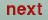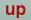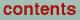Next: Hartree potential plot Up: Plot programs Previous: Common features of the

## Charge density and ELF plot

The program gnucharge.run generates plots of the calculated charge density or the Electron Localization Function (ELF). The program reads the files RHO and ELF created by lm.run in charge mode (CHARGE=T in category OPTIONS, ELF=T in category CHARGE) and creates the plot data file CHARGE.DAT and the command file CHARGE.GNU. After the selection of the output device follows the question:

`generate a charge density(t) or ELF plot(f)?`
Entering t (logical true) or / (End-of-record) selects a charge density plot, whereas f (logical false) selects a plot of the ELF. In the next step the plot data are read in and the minimum and maximum are determined:
``` Number of grid-points:  2821
the charges range from .660E-03 to .581E+01```
By default the plot range extends from the minimum to the maximum of the data. However, you can modify the range by entering two scalars bounding the new interval:
`  enter new min, max or / for default`
The following question concerns the number of isocontour lines to be drawn
` how many contours?(default is 11)`
You may enter any number between 2 and 98 or / to accept the default. The iso contours are now equally spaced from minimum to maximum including the upper and lower bound. Assuming 11 lines for a minimum of 0.0 and a maximum of 1.0 results in contours representing the values 0, 0.1, 0.2 ... , 0.9, 1.0. The next question defines the nature of the plot:
` draw a 3d-surface(t) or not(f)?(default: f)`
Entering t (logical true) places a 3d surface with hidden-line-removal on top of the contour plot, whereas f (logical false) or / lead to a simple contour plot. Now the plotfile or the plot window should be generated.

Important notice: Gnuplot does not retain proper x-y-scaling for most output formats unless your plot is quadratic. You generally have to scale the plot manually by editing the directive ''set size ...'' in CHARGE.GNU.

O. Jepsen
Thu Oct 12 14:48:45 MESZ 2000